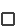# Equity Values: Discounted Cash Flow

Equity risk measured on a cash basis, reflecting the long-term relationship between dividend yields and triple-A bond yields may understate the value of equities when dividend yields are low and earnings growth is rapid.

Did extreme earnings growth justify extraordinary price-earnings ratios in the 1990s?

Equity risk measured in terms of historic price-earnings ratios may be too inflexible.

The question is, 'Can earnings growth be so attractive as to justify extraordinary price-earnings ratios?'

However, we have a third alternative: equity risk measured using the principles of John Burr Williams' present value equation for discounting projected dividends for the entire market over a number of years.

## John Burr Williams' Formula

John Burr Williams' equation for discounting the future stream of dividends takes into consideration current dividends, the investor's target rate of return, and a projected rate of dividend growth over an infinite period.

Here is the equation:

[ Value = D/(I-G) ]

Because of the Petersburg Paradox, this formula is not meaningful when growth rates exceed the investor's target rate of return.

Stock values increase with the rate of growth of dividends.

However, by limiting the scope of the formula to a number of years, William's concept that the value of equities should be proportionate to the dividend stream, taking growth into consideration, and inversely proportionate to the investor's desired rate of return, is useful.

Stock values increase with the rate of growth of dividends and decrease when investors demand higher returns.

## Discounting Macro Dividend Flows

To apply John Burr Williams' formula to the overall market, we may use total dividends paid by nonfinancial, nonfarm corporations from the Federal Reserve flow of funds accounts (Table F.213) to represent the variable 'D'.

For the growth rate, 'G', we will use the long-term (fifty-five-year) compound rate of growth of total before-tax profits of nonfinancial, nonfarm corporations, also derived from the national flow of funds accounts.

Growth of before-tax profits, rather than growth of dividends, is chosen because growth rates for dividends and after-tax profits have been distorted by declining corporate income tax rates and changes in dividend policy — trends that cannot be expected to continue indefinitely.

## Long-Term Growth

Over the period 1946-2003, the geometric average annual growth rate of before-tax profits of nonfarm nonfinancial corporations has been 5.3%.

The long-term growth of before-tax U.S. corporate profits has been 5.3%.

This rate has fluctuated wildly from year to year, but there has been no long-term improvement, as sometimes supposed.

In relation to the entire market, the rate of growth of before-tax profits represents maximum growth for dividends.

In the long-run, dividends cannot grow faster than before-tax profits and corporate tax rates cannot fall lower than zero.

## Unpredictable Dividend Policies

Current dividend payout rates may be higher or lower than what actually occurs over time, but there is no way to predict dividend payout policy for the entire market, much less relate expected payout ratios to growth.

Buybacks reduce the intrinsic value of equities for permanent investors.

Policies regarding stock buybacks in the last generation have distorted traditional dividend practices and are harmful to long-term shareholders, reducing the intrinsic value of equities for permanent investors.

Buyback policies are vulnerable to eventual change, if and when commonsense returns.

 John Burr Williams' formula values equities by taking into consideration :projected dividend growth . Over the long-term, dividend growth cannot exceed:the Federal Funds rate.long-term earnings growth.management expectations.household rate of saving. Over the period 1946-2003, average annual growth of pre-tax profits of U.S. nonfarm nonfinancial corporations was: